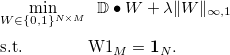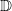# Large-Scale Exemplar Clustering (Subset Selection / Facility Location)

## Description

### Lowrank-ALBCD is a scalable convex exemplar clustering (or subset selection / facility location) solver based on Augmented Lagrangian + Block Coordinate Descent (AL-BCD) that uses column-generation technique to avoid computing the pairwise sample similarity matrix.

In particular, LowrankALBCD solves problem of the form:by convex relaxation from {0,1} to [0,1], whereis an N by M dissimilarity matrix,denotes element-wise inner product, N is the number of samples, and M is the number of candidate exemplars. In the default setting, we have M=N and normalized Euclidian distance square is used. We provide a tightening procedure for the relaxation that guarantees to yeild integer solution. (The solver by default uses normalized square Euclidean distance. To use other dissimilarity measure, send an email to eyan@cs.cmu.edu.)

## Usage

Usage: LowrankALBCD [data]
output: "clusters"
-l lambda: parameter controling number of clusters (default 0.02*#samples)
-t tightening: incrementally rounding to integer solution (0/1, default 1)
-e eta: Augmented Lagrangian parameter (default 0.01)
-m max_iter: maximum number of iterations (default 1000)
-p precision: primal infeasibility tolerence (default 1e-2)
-c cache_size: maximum number of columns of dissimilarity matrix stored (default 10000)
(p.s. Normalized square Euclidean distance d(xi,xj)=2-\frac{}{\|xi\|_2\|xj\|_2} is used by default.)


## Data Format:

LIBSVM (svm-light) format:
Each row
        [label] [feature_ID_1]:[value1] [feature_ID_2]:[value2] ......

corresponds to a sample, where [label] is the ground-truth cluster ID (can be filled in with 0 if ground truth is unknown) and features of 0 value are not listed. One can find some examples in the directory "example_data/", and more data of this format can be found at, for example LIBSVM repository.

## Output Format:

File "clusters":
Each row
        [exemplar_ID] [sample_ID1]:[assign_value1] [sample_ID2]:[assign_value2] ......

corresponds to a cluster, where [exemplar_ID] is the ID of sample selected as exemplar, which is then followed by a sequence of [sample_ID] with non-zero weight on this exemplar.

## Example Usages:

1. The default behavior of the solver uses "tightening" technique to enforce integer solution. For example, type:
	\$ ./LowrankALBCD example_data/real-sim.3k

It will solve several convex optimization problems. Each problem is obtained by rounding one more exemplar (a column of W) to integers and solve for the others.
2. For some data, for example:
	         ./LowrankALBCD example_data/german.numer.scale

The solver obtains integer solution without tightening. In this case, the solution is guaranteed to be the optimal solution to the combinatorial (exemplar clustering) problem.
3. If one would like to run convex relaxation solver without tighteing, add "-t 0" as:
	        ./LowrankALBCD -t 0 example_data/real-sim.3k

4. To find different number of clusters, one can tune the parameter "lambda" by "-l" option. For example:
	        ./LowrankALBCD -l 20 example_data/german.numer.scale
./LowrankALBCD -l 10 example_data/german.numer.scale
./LowrankALBCD -l 5 example_data/german.numer.scale
./LowrankALBCD -l 1 example_data/german.numer.scale

will gives 4, 8, 12, 53 clusters respectively.

## Citation

### You are welcome to use the code. For citation, please use:

Scalable Exemplar Clustering and Facility Location via Augmented Block Coordinate Descent with Column Generation.
Ian E.H. Yen, Dmitry Malioutov, and Abhishek Kumar. ,
In International Conference on Artificial Intelligence and Statistics (AISTATS), 2016. [PDF]

Bug reports and comments are always appreciated.